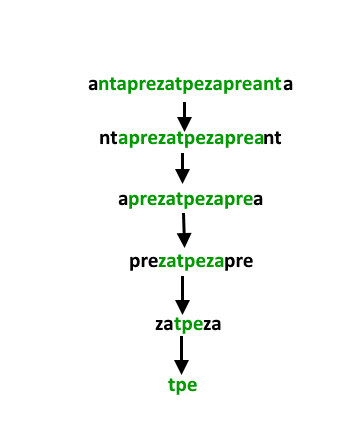Related Articles
Longest Possible Chunked Palindrome
• Difficulty Level : Medium
• Last Updated : 10 Jun, 2020

Given a string, the task is to return the length of its longest possible chunked palindrome. It means a palindrome formed by substring in the case when it is not formed by characters of the string. For a better understanding look at the example

Examples:

```Input : ghiabcdefhelloadamhelloabcdefghi
Output : 7
(ghi)(abcdef)(hello)(adam)(hello)(abcdef)(ghi)

Input : merchant
Output : 1
(merchant)

Input : antaprezatepzapreanta
Output : 11
(a)(nt)(a)(pre)(za)(tpe)(za)(pre)(a)(nt)(a)

Input : geeksforgeeks
Output : 3
(geeks)(for)(geeks)
```

## Recommended: Please try your approach on {IDE} first, before moving on to the solution.

The entire idea is to create chunks from left and right and recursively.As you can see, we can match substring from the left side chunk and match it with the exact right side chunk. Once we get a match, we recursively count the length of the longest possible chunked palindrome in the remaining string. We end the recursion once no string is left or when no more valid chunked parts can be found.

## Java

 `/* Java program to find the length of longest palindromic``   ``chunk */``import` `java.util.*;``import` `java.lang.*;``import` `java.io.*;`` ` `class` `LongestPalindromicChunk``{``    ``// Here s is the string whose LCP is needed``    ``// ln is length of string evaluated till now``    ``// and str is original string``    ``private` `static` `int` `LPCRec(String curr_str, ``int` `count,``                             ``int` `len, String str)``    ``{``        ``// if there is noting at all!!``        ``if` `(curr_str == ``null` `|| curr_str.isEmpty())``            ``return` `(``0``);`` ` `        ``// if a single letter is left out``        ``if` `(curr_str.length() <= ``1``)``        ``{``            ``if` `(count != ``0` `&& str.length() - len <= ``1``)``                ``return` `(count + ``1``);``            ``else``                ``return` `(``1``);``        ``}`` ` `        ``// for each length of substring chunk in string``        ``int` `n = curr_str.length();``        ``for` `(``int` `i = ``0``; i < n/``2``; i++)``        ``{``            ``// if left side chunk and right side chunk``            ``// are same``            ``if` `(curr_str.substring(``0``, i + ``1``).``                ``equals(curr_str.substring(n-``1``-i, n)))``            ``{``                ``// Call LCP for the part between the``                ``// chunks and add 2 to the result.``                ``// Length of string evaluated till``                ``// now is increased by (i+1)*2``                ``return` `LPCRec(curr_str.substring(i+``1``, n-``1``-i),``                           ``count + ``2``,``                           ``len + (i+``1``)*``2``, str);``            ``}``        ``}`` ` `        ``return` `count + ``1``;``    ``}`` ` `    ``// Wrapper over LPCRec()``    ``public` `static` `int` `LPC(String str)``    ``{``        ``return` `LPCRec(str, ``0``, ``0``, str);``    ``}`` ` `    ``// driver function``    ``public` `static` `void` `main(String[] args)``    ``{``        ``System.out.println(``"V : "` `+ LPC(``"V"``));``        ``System.out.println(``"VOLVO : "` `+ LPC(``"VOLVO"``));``        ``System.out.println(``"VOLVOV : "` `+ LPC(``"VOLVOV"``));``        ``System.out.println(``"ghiabcdefhelloadamhelloabcdefghi : "` `+``                        ``LPC(``"ghiabcdefhelloadamhelloabcdefghi"``));`` ` `        ``System.out.println(``"ghiabcdefhelloadamhelloabcdefghik : "` `+``                        ``LPC(``"ghiabcdefhelloadamhelloabcdefghik"``));`` ` `        ``System.out.println(``"antaprezatepzapreanta : "` `+``                        ``LPC(``"antaprezatepzapreanta"``));``    ``}``}`

## Python3

 `# Python3 program to find length of ``# longest palindromic chunk`` ` `# Here curr_str is the string whose ``# LCP is needed leng is length of ``# string evaluated till now and s  ``# is original string ``def` `LPCRec(curr_str, count, leng, s):``     ` `    ``# If there is nothing at all!! ``    ``if` `not` `curr_str:``        ``return` `0`` ` `    ``# If a single letter is left out ``    ``if` `len``(curr_str) <``=` `1``: ``        ``if` `count !``=` `0` `and` `len``(s) ``-` `leng <``=` `1``: ``            ``return` `(count ``+` `1``) ``        ``else``:``            ``return` `1`` ` `    ``# For each length of substring ``    ``# chunk in string ``    ``n ``=` `len``(curr_str) ``    ``for` `i ``in` `range``(n ``/``/` `2``): ``         ` `        ``# If left side chunk and right ``        ``# side chunk are same ``        ``if` `(curr_str[``0` `: i ``+` `1``] ``=``=` `            ``curr_str[n ``-` `1` `-` `i : n]): ``             ` `            ``# Call LCP for the part between the ``            ``# chunks and add 2 to the result. ``            ``# Length of string evaluated till ``            ``# now is increased by (i+1)*2 ``            ``return` `LPCRec(curr_str[i ``+` `1` `: n ``-` `1` `-` `i], ``                          ``count ``+` `2``, leng ``+` `(i ``+` `1``) ``*` `2``, s)``                           ` `    ``return` `count ``+` `1`` ` `# Wrapper over LPCRec() ``def` `LPC(s): ``     ` `    ``return` `LPCRec(s, ``0``, ``0``, s)`` ` `# Driver code ``print``(``"V :"``, LPC(``"V"``))``print``(``"VOLVO :"``, LPC(``"VOLVO"``))``print``(``"VOLVOV :"``, LPC(``"VOLVOV"``))``print``(``"ghiabcdefhelloadamhelloabcdefghi :"``, ``  ``LPC(``"ghiabcdefhelloadamhelloabcdefghi"``))`` ` `print``(``"ghiabcdefhelloadamhelloabcdefghik :"``,``  ``LPC(``"ghiabcdefhelloadamhelloabcdefghik"``))`` ` `print``(``"antaprezatepzapreanta :"``, ``  ``LPC(``"antaprezatepzapreanta"``))`` ` `# This code is contributed by Prateek Gupta `

## C#

 `// C# program to find length of ``// longest palindromic chunk``using` `System;`` ` `class` `GFG``{``// Here s is the string whose LCP ``// is needed ln is length of string ``// evaluated till now and str is ``// original string ``private` `static` `int` `LPCRec(``string` `curr_str, ``int` `count, ``                          ``int` `len, ``string` `str)``{``    ``// if there is noting at all!! ``    ``if` `(``string``.ReferenceEquals(curr_str, ``null``) || ``                               ``curr_str.Length == 0)``    ``{``        ``return` `(0);``    ``}`` ` `    ``// if a single letter is left out ``    ``if` `(curr_str.Length <= 1)``    ``{``        ``if` `(count != 0 && str.Length - len <= 1)``        ``{``            ``return` `(count + 1);``        ``}``        ``else``        ``{``            ``return` `(1);``        ``}``    ``}`` ` `    ``// for each length of substring ``    ``// chunk in string ``    ``int` `n = curr_str.Length;``    ``for` `(``int` `i = 0; i < n / 2; i++)``    ``{``        ``// if left side chunk and right side chunk ``        ``// are same ``        ``if` `(curr_str.Substring(0, i + 1).Equals(``            ``curr_str.Substring(n - 1 - i, n - (n - 1 - i))))``        ``{``            ``// Call LCP for the part between the ``            ``// chunks and add 2 to the result. ``            ``// Length of string evaluated till ``            ``// now is increased by (i+1)*2 ``            ``return` `LPCRec(curr_str.Substring(i + 1, (n - 1 - i) - ``                         ``(i + 1)), count + 2, len + (i + 1) * 2, str);``        ``}``    ``}`` ` `    ``return` `count + 1;``}`` ` `// Wrapper over LPCRec() ``public` `static` `int` `LPC(``string` `str)``{``    ``return` `LPCRec(str, 0, 0, str);``}`` ` `// Driver Code``public` `static` `void` `Main(``string``[] args)``{``    ``Console.WriteLine(``"V : "` `+ LPC(``"V"``));``    ``Console.WriteLine(``"VOLVO : "` `+ LPC(``"VOLVO"``));``    ``Console.WriteLine(``"VOLVOV : "` `+ LPC(``"VOLVOV"``));``    ``Console.WriteLine(``"ghiabcdefhelloadamhelloabcdefghi : "` `+ ``                    ``LPC(``"ghiabcdefhelloadamhelloabcdefghi"``));`` ` `    ``Console.WriteLine(``"ghiabcdefhelloadamhelloabcdefghik : "` `+ ``                    ``LPC(``"ghiabcdefhelloadamhelloabcdefghik"``));`` ` `    ``Console.WriteLine(``"antaprezatepzapreanta : "` `+ ``                    ``LPC(``"antaprezatepzapreanta"``));``}``}`` ` `// This code is contributed by Shrikant13`

Output:
```V : 1
VOLVO : 3
VOLVOV : 5
ghiabcdefhelloadamhelloabcdefghi : 7
ghiabcdefhelloadamhelloabcdefghik : 1
antaprezatepzapreanta : 11
```

Following is the C++ implementation with memoization for above problem.

 `#include ``#include ``#include ``using` `namespace` `std;`` ` `unordered_map mem;`` ` `int` `process(string& s, ``int` `l, ``int` `r) {``    ``int` `ans = 1;``    ``if` `(l > r)``        ``return` `0;``    ``// check if we've already solved this``    ``if` `(mem.find(s.substr(l, r-l+1)) != mem.end())``        ``return` `mem[s.substr(l, r-l+1)];``    ``for` `(``int` `len = 1; len <= (r-l+1)/2; len++) {``        ``if` `(s.substr(l, len) == s.substr(r-len+1, len))``            ``ans = max(ans, 2 + process(s, l+len, r-len));``    ``}``    ``// remember result for future``    ``mem[s.substr(l, r-l+1)] = ans;``    ``return` `ans;``}`` ` `int` `LPC(string s) {``    ``return` `process(s, 0, s.length()-1);``}`` ` `int` `main() {``    ``cout << LPC(``"aaaaabaababbaabaaababababababababbbbaaaaa"``) << endl;``    ``return` `0;``}`

Source: CareerCup

This article is contributed by Aditya Nihal Kumar Singh. If you like GeeksforGeeks and would like to contribute, you can also write an article using contribute.geeksforgeeks.org or mail your article to contribute@geeksforgeeks.org. See your article appearing on the GeeksforGeeks main page and help other Geeks.

Please write comments if you find anything incorrect, or you want to share more information about the topic discussed above.

Attention reader! Don’t stop learning now. Get hold of all the important DSA concepts with the DSA Self Paced Course at a student-friendly price and become industry ready.

My Personal Notes arrow_drop_up